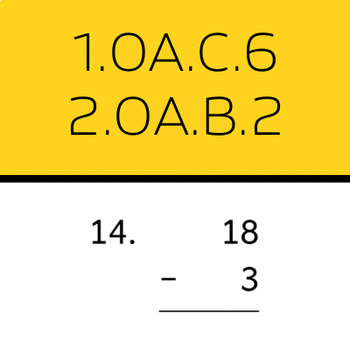# 1.OA.C.6, 2.OA.B.2: Add and subtract within 20 (50 worksheets)Subject
Resource Type
File Type

PDF

(199 KB)
Product Rating
Standards
• Product Description
• StandardsNEW
• Mixed addition and subtraction problems with sums and differences between 1 and 20.
• Contains problems in mixed horizontal and vertical orientations.
• Comprises 50 math worksheets, each with a corresponding answer page.
• CCSS: 1.OA.C.6, 2.OA.B.2.
Fluently add and subtract within 20 using mental strategies. By end of Grade 2, know from memory all sums of two one-digit numbers.
Add and subtract within 20, demonstrating fluency for addition and subtraction within 10. Use strategies such as counting on; making ten (e.g., 8 + 6 = 8 + 2 + 4 = 10 + 4 = 14); decomposing a number leading to a ten (e.g., 13 - 4 = 13 - 3 - 1 = 10 - 1 = 9); using the relationship between addition and subtraction (e.g., knowing that 8 + 4 = 12, one knows 12 - 8 = 4); and creating equivalent but easier or known sums (e.g., adding 6 + 7 by creating the known equivalent 6 + 6 + 1 = 12 + 1 = 13).
Total Pages
N/A
Included
Teaching Duration
N/A
Report this Resource to TpT
Reported resources will be reviewed by our team. Report this resource to let us know if this resource violates TpT’s content guidelines.